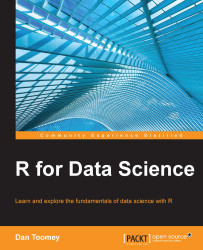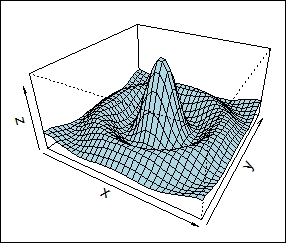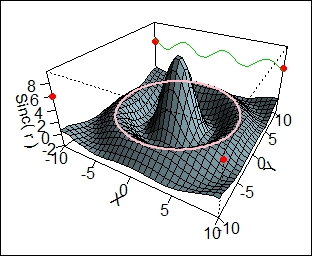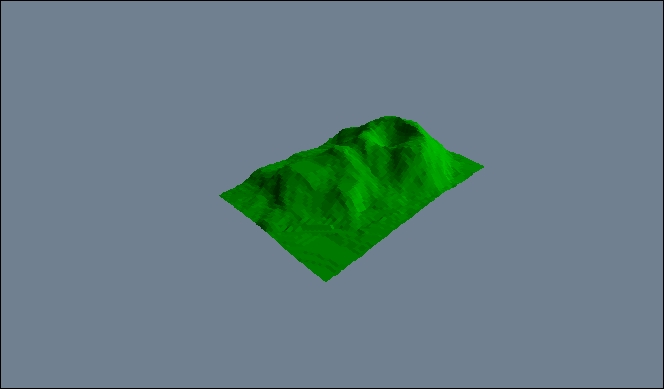•#### R for Data Science#### Overview of this book

R for Data ScienceCreditswww.PacktPub.comPrefaceFree Chapter
Data Mining PatternsData Mining SequencesText MiningData Analysis – Regression AnalysisData Analysis – CorrelationData Analysis – ClusteringData Visualization – R GraphicsData Visualization – PlottingData Visualization – 3DMachine Learning in ActionPredicting Events with Machine LearningSupervised and Unsupervised LearningIndex## Generating 3D graphics

One of the built-in functions to generate 3D graphics is `persp`. The `persp` function draws perspective plots of a surface over the x-y plane. The `persp` function has many optional parameters and will likely produce the graphics that you need. As a test, you can use the following example function, and it will generate three 3D graphics:

`> example(persp)`

This function call will generate three graphics.

(Included in the output are all of the commands necessary to generate the graphics.)

The first generated image shows a 3D plot of a rotated sine function. The associated R commands (also generated for you) are as follows:

`> persp(x, y, z, theta = 30, phi = 30, expand = 0.5, col = "lightblue")`

Here's the first generated image:The next image is a more detailed view of the same data:Lastly, we have a simulated 3D surface area presentation:The `persp` function looks like this:

```persp(x = seq(0, 1, length.out = nrow(z)),
y = seq(0, 1, length.out = ncol(z)),
z, xlim = range...```